Latest Banking jobs   »   Quantitative Aptitude Quiz For RBI Grade...

# Quantitative Aptitude Quiz For RBI Grade B Phase 1 2023 – 5th February

Direction (1–5): What will come in the place of question (?) mark in following the question: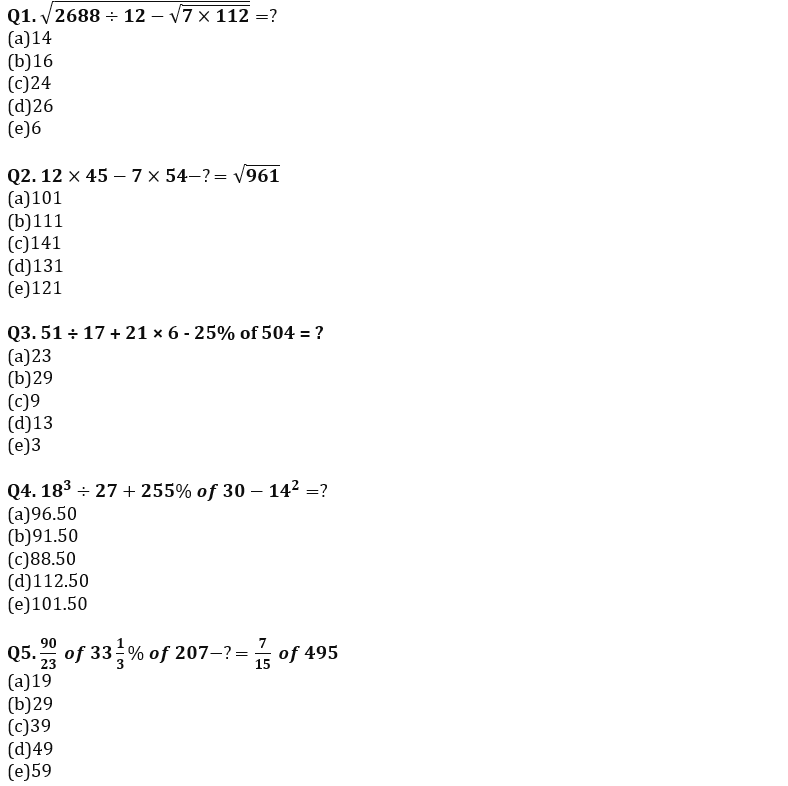Q6. Veer is 40% less efficient than Bhavya and both complete the work together in 30 days, if Abhishek takes 10 days less as Veer and Bhavya takes together. Then find how many days will be required to complete the work, if all three work together?
(a) 16 days
(b) 12 days
(c) 10 days
(d) 8 days
(e) 18 days

Q7. Sameer borrowed 17500 Rs. from Divyaraj on compound interest annually at the rate of 20% per annum, if he paid 5000 Rs. at the end of every year to Divyaraj then find how much amount Sameer have to pay at the end of fourth year for complete his debt?
(a) 14168
(b) 14648
(c) 14848
(d) 14448
(e) 14248

Q8. Ankit gets 6000 Rs. out of total profit of 9000 Rs. and he invested 8000 more amount than his partner for 8 months and his partner Arun invested his amount for whole the year in partnership, then find how much amount invested by Ankit?
(a) 8000 Rs.
(b) 1000 Rs.
(c) 9000 Rs.
(d) 14000 Rs.
(e) 12000 Rs.

Q9. Cost price of a table is 35% more than cost price of a chair, if shopkeeper sold chair at 25% profit and table at 33⅓% of loss he gets the total loss of 1350 Rs. find the cost price of table?
(a) 9012.5 Rs.
(b) 9112.5 Rs.
(c) 9212.5 Rs.
(d) 9002.5 Rs.
(e) 9122.5 Rs.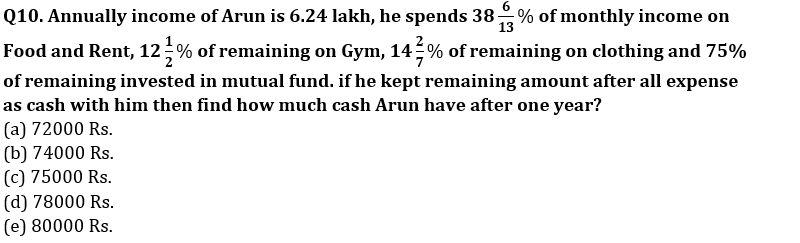Q11.How many arrangements can be formed out of the letters of the word ‘EXAMINATION’ so that vowels always occupy odd places?
(a) 21600
(b) none of these
(c) 32400
(d) 10800
(e)5400

Q12. The average age of the students in a class is 40yrs and if a student whose age was 30 yrs is absent from the class, then average age of those student who were present goes up by 2. How many students were there in the class initially?
(a) 10
(b) 6
(c) 8
(d) 12
(e)15

Q13.RAVI KANT SHARMA is thrice as efficient as Ayush and hence completes a work in 60 days less than the numbers of days taken by Ayush to complete the work. What will be the number of days taken by both of them to complete the work together?(in days)
(a) 22.5
(b) 30
(c) 60
(d) 37.5
(e)45

Q14. Maanik has. a total of 20 red and blue marbles in two bags (each bag has marbles of both colors). A marble is randomly drawn from the first bag followed by another randomly drawn from the second bag, the probability of both being red is 5/16. What is the probability of both marbles being blue?
(a) 5/16
(b) 7/18
(c) none of these
(d) can’t be determined
(e) 3/16

Q15. Four year ago, the ratio of the ages of A and B was 13:9 and eight year hence it would be 4:3. The difference of their ages four year hence will be?(in years)
(a) 15
(b) 10
(c) 12
(d) 16
(e)20

Solutions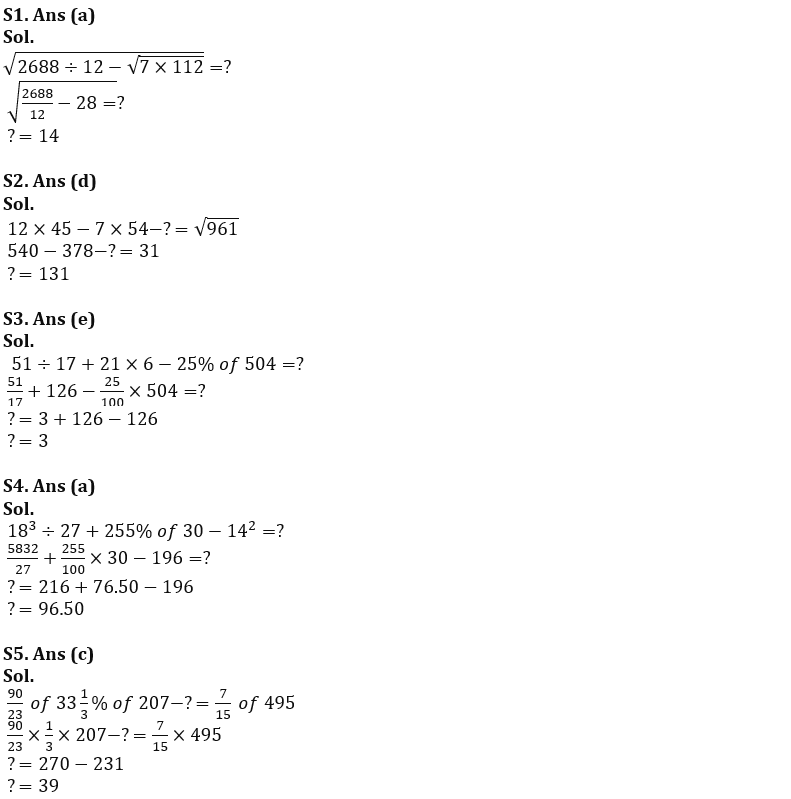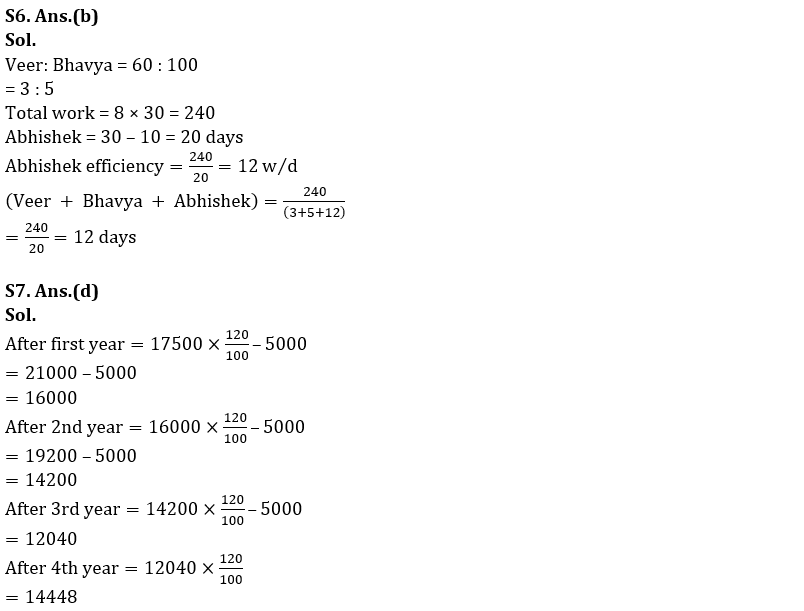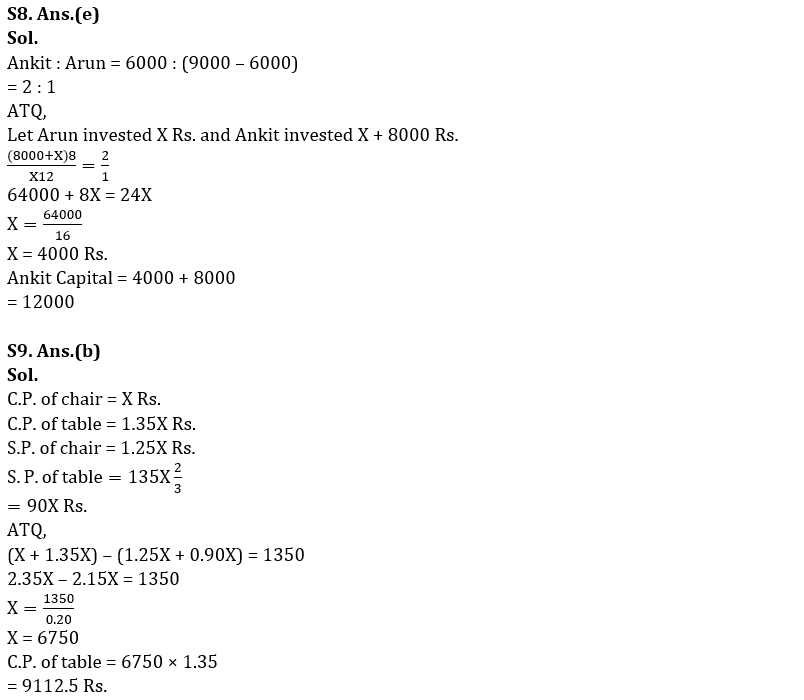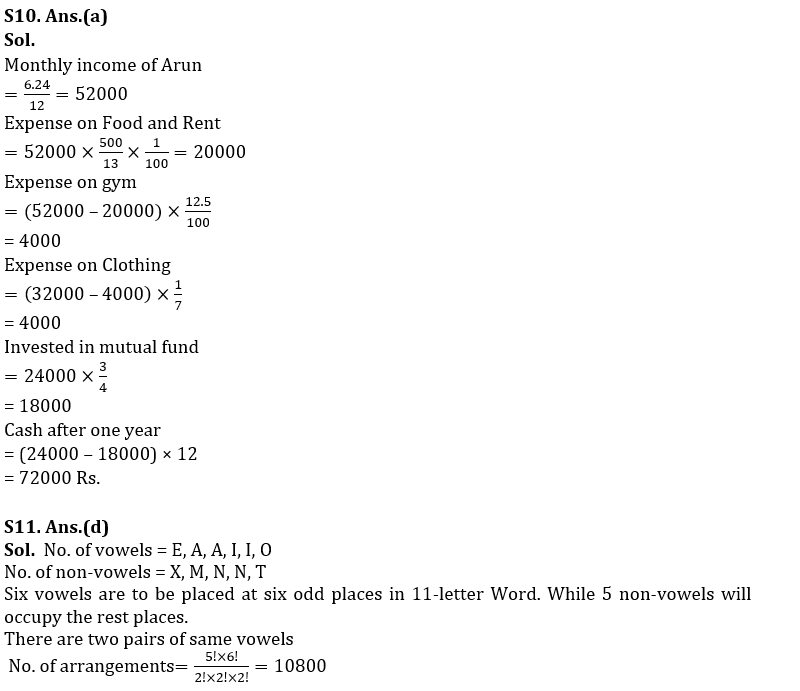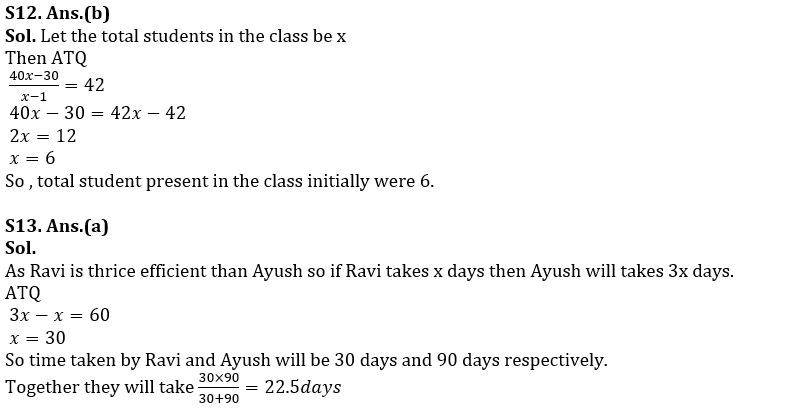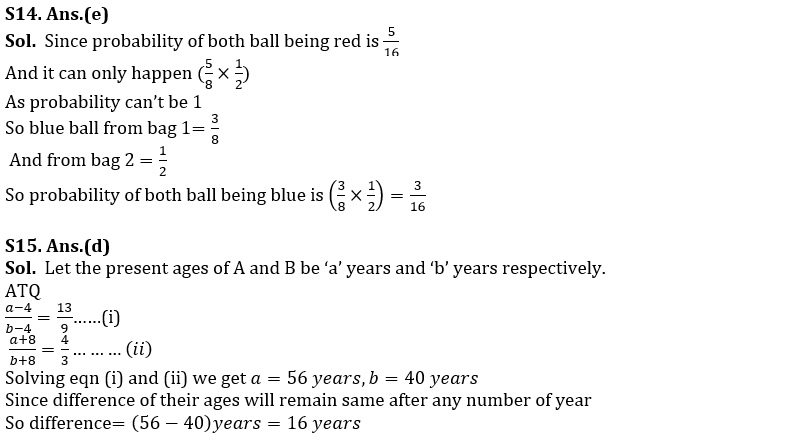## FAQs

### Quantitative Aptitude Quiz For RBI Grade B Phase 1 2023-3rd February

There are 4 sections in the RBI Grade B Phase 1 Exam i.e. English Language, General Awareness, Quantitative Aptitude & Reasoning.

#### Congratulations!Union Budget 2023-24: Free PDF